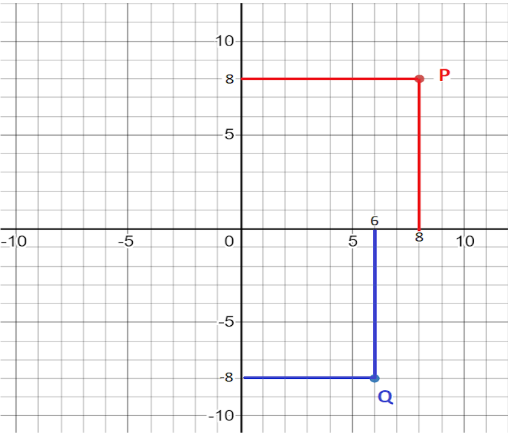Courses
Courses for Kids
Free study material
Free LIVE classes
MoreLIVE
Join Vedantu’s FREE Mastercalss

# State the abscissa and ordinate of the following point and describe the position of each point$\left( i \right)P\left( {8,8} \right) \\ \left( {ii} \right)P\left( {6, - 8} \right) \\$Verified
361.5k+ views
Hint: Project the points on the cartesian plane. From the point draw lines perpendicular to the axes and calculate the distance from origin.

Given the two points:
$P\left( {8,8} \right) \\ Q\left( {6, - 8} \right) \\$
We need to find the abscissa and ordinate of the above points and describe the position of each point.
Abscissa is the distance from a point to the vertical or y -axis, measured parallel to the horizontal or x -axis. It is also known as the x -coordinate.
Ordinate is the distance from a point to the horizontal or x -axis, measured parallel to the vertical or y -axis. It is also known as the y -coordinate.
Now we plot the point on the cartesian plane. This describes the position of points.From the cartesian plane, by projecting the point (A) and (B) on x and y axes, we get the following data.
The distance of point (P) to the vertical or y -axis, measured parallel to the horizontal or x -axis is 8 units. Hence abscissa for point (P) is 8 units.
The distance of point (P) to the horizontal or x -axis, measured parallel to the vertical or y -axis is 8 units. Hence the ordinate for point (P) is 8 units.
Similarly, the distance of point (Q) to the vertical or y -axis, measured parallel to the horizontal or x -axis is 6 units. Hence abscissa for point (Q) is 6 units.
And the distance of point (Q) to the horizontal or x -axis, measured parallel to the vertical or y -axis is 8 units. Since it lies in the fourth quadrant, the ordinate for point (Q) is -8 units.
Therefore, for point $P\left( {8,8} \right)$,abscissa = 8 units and ordinate = 8 units, for point $Q\left( {6, - 8} \right)$ , abscissa = 6 units and ordinate = -8 units.

Note: For the problems like above the points should always be projected on the cartesian plane. The sign of the abscissa and ordinate depends upon the quadrant in which the point lies in the cartesian plane.
Last updated date: 27th Sep 2023
Total views: 361.5k
Views today: 5.61k